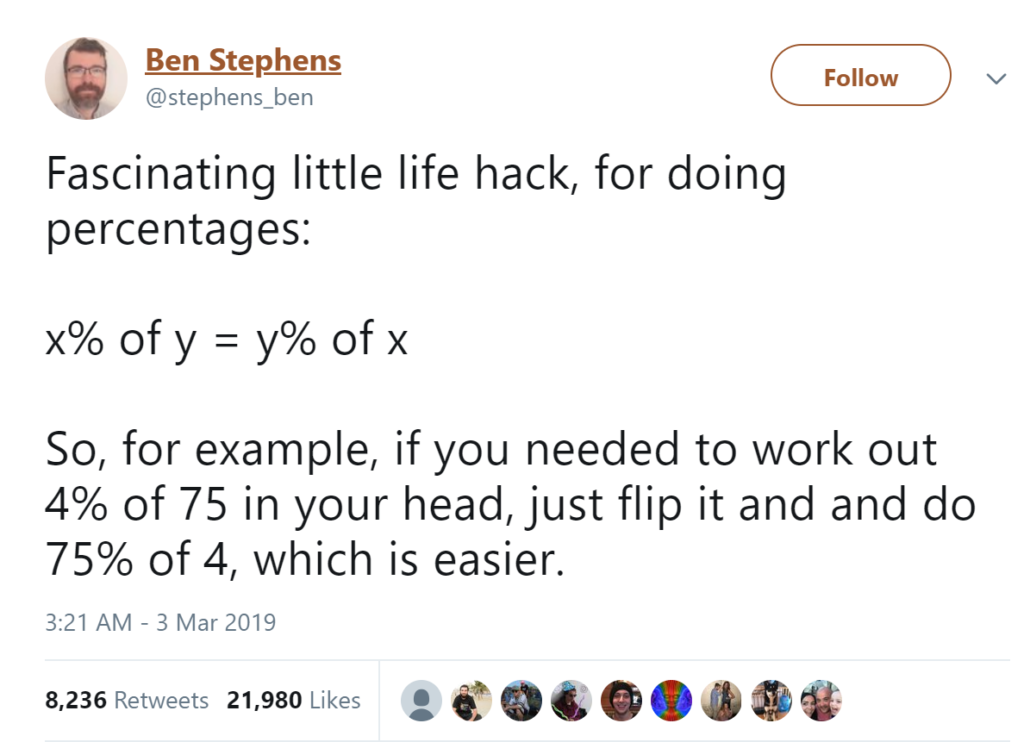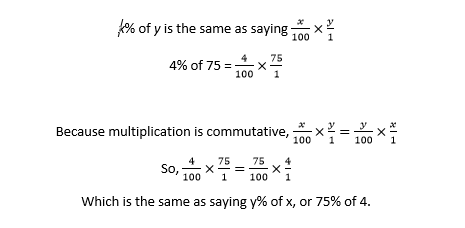# The New Viral Math Trick That Isn’t Very New

A useful math trick that could save you tons of time and mental effort has sped across the Internet, leaving half the population wondering why they weren’t taught this in school and the other half of the population wondering why so many people are flabbergasted by something they thought everyone already knew.

Whether you’ve known this trick since your elementary days or you’re just now learning it when you’re today years old, let’s review this nifty trick for quickly calculating percentages.It doesn’t just work with 4 and 75.

Quick: What’s 28% of 25?

28% of 25 is 25% of 28. 25% is ¼, so we divide 28 by 4 to get 7. 28% of 25 is 7. Boom.

So why does it work? In math-speak, it works because multiplication is commutative. In non-math-speak, that means the order of the numbers in a multiplication problem doesn’t change the answer, so a × b is the same as b × a. Let’s take a look:Voila!

These kinds of math tricks make so much sense once someone shows you the mechanics of how they work. Our math tutors specialize in teaching students the kinds of math “hacks” that make math simple. If you’d like a free math tutoring consultation, contact your local center today!

Tags: